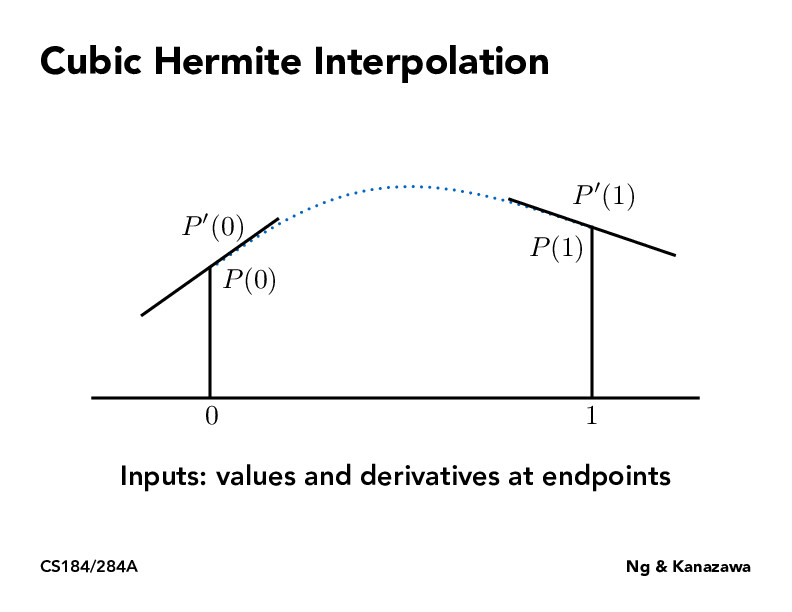Lecture 7: Splines and Bezier Curves (27)han20192019

I think the idea behind cubic hermite interpolation is that in order to specify a cubic polynomial, we need four conditions. So the four conditions are P(0), P(1) and the derivative at 0 and 1. The catmull-roll space curve is similar and it calculate the derivative based on the four points given. So my question is that what's the difference between cubic hermite interpolation and catmull-roll space curve? Don't they all use two points and two derivatives?wcyjames

@han20192019: After watching the lecture, I think the Catmull-Rom space curve uses cubic Hermite interpolation essentially, while the two derivatives of the four inputs are also linearly interpolated in Catmull-Rom space curve.

You must be enrolled in the course to comment